# Differentiating Inverse Trigonometric Functions

## Overview

An inverse function is a function that reverses the effect of applying the function for which it is the inverse. For example, the square function reverses the effect of the square root function, and vice versa:

y  =  √x,   y 2  =  x

Stating this a little more formally, two functions ƒ(x) and g(x) are the inverse of one another if:

ƒ(g(x))  =  x   and   g(ƒ(x))  =  x

It can be shown that there is also a relationship between the derivatives of two functions ƒ(x) and g(x) that are the inverse of one another, which we can write as:

 g′(x)  = 1 ƒ′(g(x))

If you have studied trigonometry (you should at least have a reasonable grasp of the basics in order to study calculus) you will be aware that there are six commonly used trigonometric functions. They are the sine, cosine, tangent, secant, cosecant and cotangent functions. You should also be aware that each of these functions has a corresponding inverse trigonometric function. These are (respectively) the arcsine, arccosine, arctangent, arccotangent, arcsecant and arccosecant functions.

Each of the six inverse trigonometric functions reverses the effect of the corresponding trigonometric function. For example, if we apply the sine function to an angle, we get the sine of that angle as the output. The inverse of the sine function is the arcsine function. If we take the sine of an angle and apply the arcsine function to it, the output will be the original angle. Actually that's not entirely true. Of the six trigonometric functions, only the sine and cosine function are continuous, but all of the trigonometric functions are periodic. That means they repeatedly produce the same set of output values over regular intervals. For most of the trigonometric functions, that interval is . The exceptions are the tangent and cotangent functions, for which the interval is π.

As a consequence of their periodicity, each trigonometric function will produce the same output value for an infinite number of different input values. That causes a problem since, because applying the inverse function to this output will produce only one value. This means that our definition of the relationship between two functions that are the inverse of each other does not work. As an example, if you apply the sine function to the angle π/2 radians (45°), you get 0.707 as the output. If you then apply the sine function to the angle 3/2π radians (135°), you get exactly the same result. Obviously, applying the arcsine function to 0.707 can only produce one output, which will be π/2 radians (45°).

In order to get around this problem, we need to restrict the domain of each of the six trigonometric functions so we have a one-to-one relationship between the input and output values for each function. In other words, we choose a domain for each function so that no two different input values passed to the function can result in the same output.

In this page we will attempt to explain how the formula for finding the derivative of each of the inverse trigonometric functions has been derived. The general procedure is pretty much the same in each case. We'll start with the arcsine function.

## The arcsine function

The arcsine function is the inverse of the sine function. The sine function takes an angle as its input and gives the sine value of the angle as its output. Providing the angle lies within the sine function's restricted domain, applying the arcsine function to its sine value will give us the original angle. The following illustration shows the graph of the sine function. As you can see, the graph is a continuous waveform, and has a period of radians (360°).The graph of the sine function

For the arcsine function to be a true inverse function of the sine function, the following statement must be true:

sin (arcsin (x))  =  x   and   arcsin (sin (x))  =  x

We ensure this by restricting the domain of the function to the closed interval [-π/2, π/2], as you can see from the illustration. The function's value increases over this domain as the value of x increases, and every input value produces a unique output value. The sine function is now defined as follows:

y  =  sin (x)   for   -π/2xπ/2

Let's see how the derivative of the arcsine function is obtained. Remember that we want to differentiate the arcsine function in respect of x, so what we are trying to find is:

 d (arcsin (x)) dx

The first step is to set y equal to the arcsine function:

y  =  arcsin (x)   for   -π/2yπ/2

However, the sine function is the inverse of the arcsine function so we can rewrite this as:

sin (y)  =  x

We are now going to use implicit differentiation, and solve for dy/dx in order to get the derivative of the arcsine function in terms of y (remember, our ultimate goal is to get the derivative of the arcsine function in terms of x, but this step takes us closer to that goal). First we need to differentiate both sides of the equation:

 d (sin(y))  = d (x) dx dx

Differentiating the right-hand side of the equation is easy enough. When differentiating the left-hand side however, we should remember that y is a function of x, so sin (y) is actually a composite function. That means that we must apply the chain rule. The chain rule tells us that the derivative of a composite function is obtained by multiplying the derivative of the outer function by the derivative of the inner function. In this case, the outer function is sin (y) and the inner function is y(x), so we get:

 cos (y)) · dy =  1 dx

Let's rearrange this to get dy/dx on its own on the left hand side:

 dy = 1 dx cos(y)

Since we know that y is equal to arcsin (x), we can substitute for y on the left-hand side of this equation as follows:

 d (arcsin (x))  = 1 dx cos (y)

We now have a formula (of sorts) for the derivative of the arcsine function, but we still need to rewrite the right-hand side of the equation in terms of x. This essentially means we need to replace the term cos (y) with some term that only involves x. Help is at hand in the form of the trigonometric identity known as the Pythagorean identity, which essentially gives us Pythagoras' theorem in trigonometric terms. The Pythagorean identity states:

cos 2 (y) + sin 2 (y)  =  1

The variable y in the Pythagorean identity represents one of the internal angles of a right-angled triangle. It is also the angle we are inputting to the sine function. Remember also that we have already established that sin (y) is equal to x. If we substitute x for sin (y) in the Pythagorean identity, we get:

cos 2 (y) + x 2  =  1

From this we get:

cos (y)   =  √(1 - x 2)

We can now rewrite our formula for the derivative of the arcsine function and get it into the required format by substituting for cos (y) as follows:

 d (arcsin (x))  = 1 dx √(1 - x 2)

The illustration below shows the graph of the arcsine function over its entire domain, which is the closed interval [-1, 1]. Note that the function can only be differentiated on the open interval (-1, 1). An open interval, remember, does not include the end points. Although both minus one and one are included in the domain of the arcsine function, the function is not differentiable for these values of x. Try plugging either of them into the derivative formula and you'll see why - you will get zero in the denominator. The tangent to the graph at either of these points will be a vertical line, so the slope is said to be undefined.The graph of y = arcsin (x) for - π/2yπ/2

## The arccosine function

The arccosine function is the inverse of the cosine function. The cosine function takes an angle as its input and gives the cosine value of the angle as its output. Providing the angle lies within the cosine function's restricted domain, applying the arccosine function to its cosine value will give us the original angle. The following illustration shows the graph of the cosine function. As with the sine function, the graph is a continuous waveform, and has a period of radians (360°). The cosine function achieves its maximum value π/2 radians ahead of the sine function, and is said to lead the sine function by π/2 radians (90°).The graph of the cosine function

For the arccosine function to be a true inverse function of the sine function, the following statement must be true:

cos (arccos (x))  =   x   and   arccos (cos (x))  =  x

We ensure this by restricting the domain of the function to the closed interval [0, π], as you can see from the illustration. The function's value decreases over this domain as the value of x increases, and every input value produces a unique output value. The cosine function is now defined as follows:

y  =  cos (x)   for   0 ≤ x ≤ π

Let's see how the derivative of the arccosine function is obtained. Remember that we want to differentiate the arccosine function in respect of x, so what we are trying to find is:

 d (arccos(x)) dx

The first step is to set y equal to the arccosine function:

y  =  arccos (x)   for   0 ≤ y ≤ π

However, the cosine function is the inverse of the arccosine function, so we can rewrite this as:

cos (y)  =  x

We will use implicit differentiation and solve for dy/dx in order to get the derivative of the arccosine function in terms of y. Differentiating both sides of the equation, we get:

 d (cos(y))  = d (x) dx dx

Remember that cos (y) is a composite function, so we need to apply the chain rule. The outer function is cos (y) and the inner function is y(x), so we get:

 -sin (y) · dy =  1 dx

Let's rearrange this to get dy/dx on its own on the left hand side:

 dy = -1 dx sin y

Since we know that y is equal to arccos (x), we can substitute for y on the left-hand side of this equation as follows:

 d (arccos(x))  = -1 dx sin y

Now we just need to rewrite the right-hand side of the equation in terms of x. The Pythagorean identity comes to our assistance once more. Just to recap, the Pythagorean identity gives us the following:

cos 2 (y) + sin 2 (y)  =  1

We have already established that cos (y) is equal to x. Substituting x for cos (y) in the Pythagorean identity gives us:

x 2 + sin 2 (y)  =  1

From this we get:

sin (y)  =  √(1 - x 2)

We can now rewrite our formula for the derivative of the arccosine function and get it into the required format by substituting for sin (y), as follows:

 d (arccos(x))  = -1 dx √(1 - x 2)

You may have noticed that this formula is almost identical to the one we obtained for the arcsine function. The only difference is the negative sign in the numerator. The illustration below shows the graph of the arccosine function over its entire domain, which is again the closed interval [-1, 1]. As with the arcsine function, both minus one and one are included in the domain of the arccosine function, but the function is not differentiable for these values of x. The tangent to the graph at either of its endpoints will be a vertical line, and the slope is said to be undefined.The graph of y = arccos (x) for 0 ≤ y ≤ π

## The arctangent function

The arctangent function is the inverse of the tangent function. The tangent function takes an angle as its input and gives the tangent value of the angle as its output. Providing the angle lies within the tangent function's restricted domain, applying the arctangent function to its tangent value will give us the original angle. The following illustration shows the graph of the tangent function. The tangent function differs from the sine and cosine functions in that its graph is not a continuous waveform, and it has a period of only π radians (180°).The graph of the tangent function

For the arctangent function to be a true inverse function of the tangent function, the following statement must be true:

tan (arctan (x))  =  x   and   arctan (tan (x))  =  x

We ensure this by restricting the domain of the function to the open interval (-π/2, π/2), as you can see from the illustration (note that the tangent function is not defined for either x = -π/2 or x = π/2). The function's value increases over this domain as the value of x increases, and every input value produces a unique output value. The tangent function is now defined as follows:

y  =  tan (x)   for   -π/2 < x < π/2

Let's see how the derivative of the arctangent function is obtained. As before, we want to differentiate the arctangent function in respect of x, so what we are trying to find is:

 d (arctan(x)) dx

The first step is to set y equal to the arctangent function:

y  =  arctan (x)   for   -π/2 < y < π/2

However, the tangent function is the inverse of the arctangent function, so we can rewrite this as:

tan (y)  =  x

We will use implicit differentiation and solve for dy/dx in order to get the derivative of the arctangent function in terms of y. Differentiating both sides of the equation, we get:

 d (tan(y))  = d (x) dx dx

Remember that tan (y) is a composite function, so we need to apply the chain rule. The outer function is tan (y) and the inner function is y(x), so we get:

 sec 2 (y) · dy =  1 dx

Let's rearrange this to get dy/dx on its own on the left hand side:

 dy = 1 dx sec 2 (y)

Since we know that y is equal to arctan (x), we can substitute for y on the left-hand side of this equation as follows:

 d (arctan (x))  = 1 dx sec 2 (y)

Now we need to rewrite the right-hand side of the equation in terms of x. This time, we won't use the Pythagorean identity, but we will be using a trigonometric identity that is closely related to it. Dividing the Pythagorean identity by cos 2 (y) gives us the following trigonometric identity:

tan 2 (y) + 1  =  sec 2 (y)

We have already established that tan (y) is equal to x, so we can rewrite this new identity as:

x 2 + 1  =  sec 2 (y)

We can now rewrite our formula for the derivative of the arctangent function and get it into the required format by substituting for sec 2 (y), as follows:

 d (arctan(x))  = 1 dx x 2 + 1

The illustration below shows the graph of the arctangent function. Whereas the domains of the arcsine and arccosine functions are both restricted to the interval [-1, 1], the domain of the arctangent function consists of all of the real numbers. The function is also differentiable throughout its entire domain.The graph of y = arctan (x) for -π/2 < y < π/2

## The arccotangent function

The arccotangent function is the inverse of the cotangent function. The cotangent function takes an angle as its input and returns the cotangent value of the angle as its output. Providing the angle lies within the cotangent function's restricted domain, applying the arccotangent function to its cotangent value will give us the original angle. The following illustration shows the graph of the cotangent function. As with the tangent function, the graph of the cotangent function is not a continuous waveform, and has a period of only π radians (180°).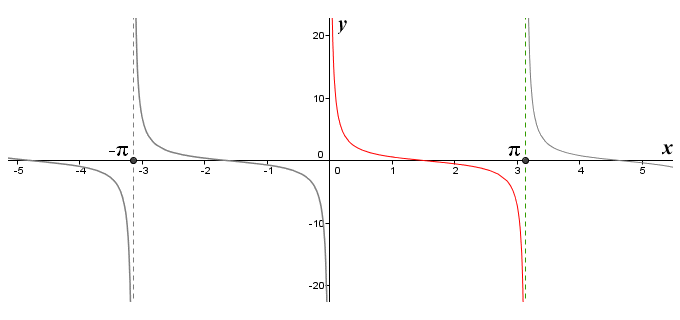The graph of the cotangent function

For the arccotangent function to be a true inverse function of the cotangent function, the following statement must be true:

cot (arccot (x))  =  x   and   arccot (cot (x))  =  x

We ensure this by restricting the domain of the function to the open interval (0, π), as you can see from the illustration (note that the cotangent function is not defined for either x = 0 or x = π). The function's value decreases over this domain as the value of x increases, and every input value produces a unique output value. The cotangent function is now defined as follows:

y  =  cot (x)   for   0 < x < π

Let's see how the derivative of the arccotangent function is obtained. We want to differentiate the arccotangent function in respect of x, so what we are trying to find is:

 d (arccot (x)) dx

The first step is to set y equal to the arccotangent function:

y  =  arccot (x)   for   0 < y < π

However, the cotangent function is the inverse of the arccotangent function, so we can rewrite this as:

cot (y)  =  x

We will use implicit differentiation and solve for dy/dx in order to get the derivative of the arccotangent function in terms of y. Differentiating both sides of the equation, we get:

 d (cot(y))  = d (x) dx dx

Remember that cot (y) is a composite function, so we need to apply the chain rule. The outer function is cot (y) and the inner function is y(x), so we get:

 csc 2 (y) · dy =  1 dx

Let's rearrange this to get dy/dx on its own on the left hand side:

 dy = -1 dx csc 2 (y)

Since we know that y is equal to arccot (x), we can substitute for y on the left-hand side of this equation as follows:

 d (arccot (x))  = -1 dx csc 2 (y)

Now we need to rewrite the right-hand side of the equation in terms of x. This time we will use another trigonometric identity that is closely related to the Pythagorean identity. Dividing the Pythagorean identity by sin 2 (y) gives us the following trigonometric identity:

cot 2 (y) + 1  =  csc 2 (y)

We have already established that cot (y) is equal to x, so we can rewrite this new identity as:

x 2 + 1  =  csc 2 (y)

We can now rewrite our formula for the derivative of the arctangent function and get it into the required format by substituting for csc 2 (y), as follows:

 d (arccot (x))  = -1 dx x 2 + 1

The illustration below shows the graph of the arccotangent function. As with the arctangent, the domain of the arccotangent function consists of all of the real numbers, and it is differentiable throughout its entire domain. You may also have noticed that the derivative of the arccotangent function is the negative of the derivative of the arctangent function.The graph of y = arccot (x) for 0 < y < π

## The arcsecant function

The arcsecant function is the inverse of the secant function. The secant function takes an angle as its input and returns the secant value of the angle as its output. Providing the angle lies within the secant function's restricted domain, applying the arcsecant function to its secant value will give us the original angle. The following illustration shows the graph of the secant function. The graph of the secant function is not a continuous waveform, as you can see. It has a period of radians (360°).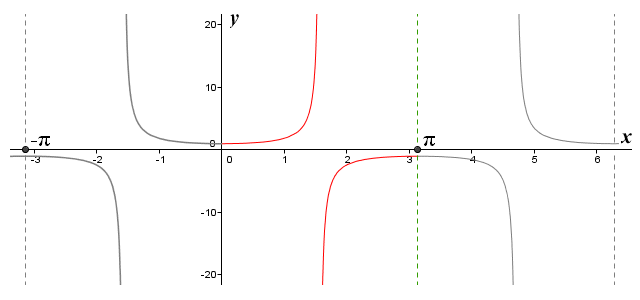The graph of the secant function

For the arcsecant function to be a true inverse function of the secant function, the following statement must be true:

sec (arcsec (x))  =  x   and   arcsec (sec (x))  =  x

We ensure this by restricting the domain of the function to the closed interval [0, π], as you can see from the illustration. The function value will increase as x increases, from one at x = 0 to some number approaching infinity as x gets closer to π/2. The function value then switches sign, but continues to increase as x increases from some number approaching negative infinity close to x = π/2 to minus one at x = π. Every input value produces a unique output value, but note that the function is not defined for x = π/2. The secant function is defined as follows:

y  =  sec (x)   for   0 ≤ x ≤ π,   except   x  =  π/2

Let's see how the derivative of the arcsecant function is obtained. As always, we want to differentiate the arcsecant function in respect of x, so what we are trying to find is:

 d (arcsec (x)) dx

The first step is to set y equal to the arcsecant function:

y  =  arcsec (x)   for   0 ≤ y ≤ π,   except   y  =  π/2

However, the secant function is the inverse of the arcsecant function, so we can rewrite this as:

sec (y)  =  x

We will use implicit differentiation and solve for dy/dx in order to get the derivative of the arcsecant function in terms of y. Differentiating both sides of the equation, we get:

 d (sec(y))  = d (x) dx dx

Remember that sec (y) is a composite function, so we need to apply the chain rule. The outer function is sec (y) and the inner function is y(x), so we get:

 sec(y) · tan(y) · dy =  1 dx

Let's rearrange this to get dy/dx on its own on the left hand side:

 dy = 1 dx sec(y) · tan(y)

Since we know that y is equal to arcsec (x), we can substitute for y on the left-hand side of this equation as follows:

 d (arcsec (x))  = 1 dx sec(y) · tan(y)

Now we need to rewrite the right-hand side of the equation in terms of x. This looks more complicated than it actually is, because we now have the product of two different trigonometric functions in the denominator on the right-hand side, both taking y as an argument. We can however still use a trigonometric identity - the same one, in fact, that we used to help us obtain the derivative of the arctangent function – to solve the problem. You may recall that if we divide the Pythagorean identity by cos 2 (y), we get the following trigonometric identity:

tan 2 (y) + 1  =  sec 2 (y)

We have already established that sec (y) is equal to x, so we can rewrite this new identity as:

tan 2 (y) + 1  =  x 2

Substituting for tan (y) in our equation, we get:

 d (arcsec(x))  = 1 dx sec (y) √( sec 2 (y) - 1)

But since we know that sec (y) is equal to x, we can rewrite this as:

 d (arcsec(x))  = 1 dx |x| √(x 2 - 1)

Note that x 2 - 1 can never evaluate to a negative number, since the arcsecant function takes the output from the secant function as its input, and as we have seen the value of sec (x) will always be ≤ -1 or ≥ 1. Note also that we use |x| in the denominator rather than x in order to ensure that dy/dx will always be positive (we can actually justify this using trigonometry, but we won't go into the details here).

The illustration below shows the graph of the arcsecant function, and you should be able to see that the derivative (which is the slope of the tangent to the graph at any point, just to remind you!) will always be positive. The domain of the function consists of all of the real numbers except for the open interval (-1, 1), and the function is differentiable for all values of x except for the closed interval [-1, 1]. Although both minus one and one are included in the domain of the arcsecant function, the function is not differentiable for these values of x. Try plugging either of them into the derivative formula and you'll see why - you will get zero in the denominator. The tangents to the graph at either of these points will be a vertical line, and their slope is said to be undefined.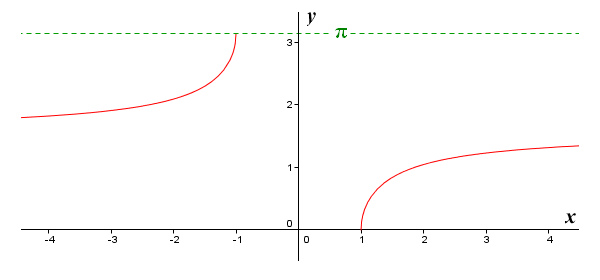The graph of y = arcsec (x) for 0 ≤ y ≤ π, except y = π/2

## The arccosecant function

The arccosecant function is the inverse of the cosecant function. The cosecant function takes an angle as its input and returns the cosecant value of the angle as its output. Providing the angle lies within the cosecant function's restricted domain, applying the arccosecant function to its cosecant value will give us the original angle. The following illustration shows the graph of the cosecant function. The graph of the cosecant function is not a continuous waveform, as you can see. It has a period of radians (360°).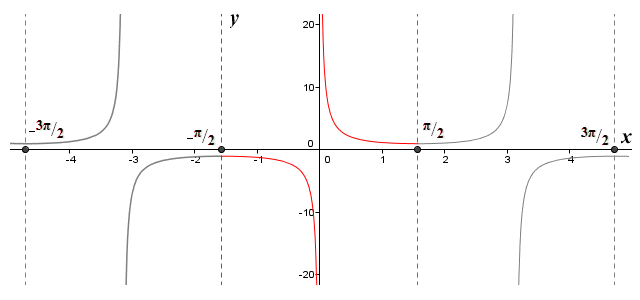The graph of the cosecant function

For the arccosecant function to be a true inverse function of the cosecant function, the following statement must be true:

csc (arccsc (x))  =  x   and   arccsc (csc (x))  =  x

We ensure this by restricting the domain of the function to the closed interval [-π/2, π/2], as you can see from the illustration. The function value will decrease as x increases, from minus one at x = -π/2 to some number approaching minus infinity as x gets closer to 0. The function value then switches sign, but continues to decrease as x increases from some number approaching infinity close to 0 to one at x = π/2. Every input value produces a unique output value, but note that the function is not defined for x = 0. The cosecant function is defined as follows:

y  =  csc (x)   for   -π/2xπ/2,   except   x = 0

Let's see how the derivative of the arccosecant function is obtained. As always, we want to differentiate the arccosecant function in respect of x, so what we are trying to find is:

 d (arccsc (x)) dx

The first step is to set y equal to the arccosecant function:

y  =  arccsc (x)   for   -π/2yπ/2,   except   x = 0

However, the cosecant function is the inverse of the arccosecant function, so we can rewrite this as:

csc (y)  =  x

We will use implicit differentiation and solve for dy/dx in order to get the derivative of the arccosecant function in terms of y. Differentiating both sides of the equation, we get:

 d (csc(y))  = d (x) dx dx

Remember that csc (y) is a composite function, so we need to apply the chain rule. The outer function is csc (y) and the inner function is y(x), so we get:

 -csc(y) · cot(y) · dy =  1 dx

Let's rearrange this to get dy/dx on its own on the left hand side:

 dy = -1 dx csc(y) · cot(y)

Since we know that y is equal to arccsc (x), we can substitute for y on the left-hand side of this equation as follows:

 d (arccsc (x))  = -1 dx csc(y) · cot(y)

Now we need to rewrite the right-hand side of the equation in terms of x. Again, this looks more complicated than it actually is, because we have the product of two different trigonometric functions in the denominator on the right-hand side, both taking y as an argument. As with the arcsecant function, we can use a trigonometric identity we have already seen to help us solve the problem. It's the same one we used to help us obtain the derivative of the arccotangent function. You may recall that if we divide the Pythagorean identity by sin 2 (y), we get the following trigonometric identity:

cot 2 (y) + 1  =  csc 2 (y)

We have already established that csc (y) is equal to x, so we can rewrite this new identity as:

cot 2 (y) + 1  =  x 2

Substituting for cot (y) in our equation, we get:

 d (arccsc(x))  = -1 dx csc (y) √( csc 2 (y) - 1)

But since we know that csc (y) is equal to x, we can rewrite this as:

 d (arccsc(x))  = -1 dx |x| √(x 2 - 1)

Note that x 2 - 1 can never evaluate to a negative number, since the arccosecant function takes the output from the cosecant function as its input, and as we have seen the value of sec (x) will always be ≤ -1 or ≥ 1. Note also that we again use |x| in the denominator rather than x in order to ensure that dy/dx will always be negative (again, this can be justified using trigonometry, but we won't go into the details here).

The illustration below shows the graph of the arccosecant function, and you should be able to see that the derivative (i.e. the slope of the tangent to the graph at any point) will always be negative. The domain of the function consists of all of the real numbers except for the open interval (-1, 1), and the function is differentiable for all values of x except for the closed interval [-1, 1]. Although both minus one and one are included in the domain of the arccosecant function, the function is not differentiable for these values of x. Try plugging either of them into the derivative formula and you'll see why - you will get zero in the denominator. The tangents to the graph at either of these points will be a vertical line, and their slope is said to be undefined. You may also have noticed that the derivative of the arccosecant function is the negative of the derivative of the arcsecant function.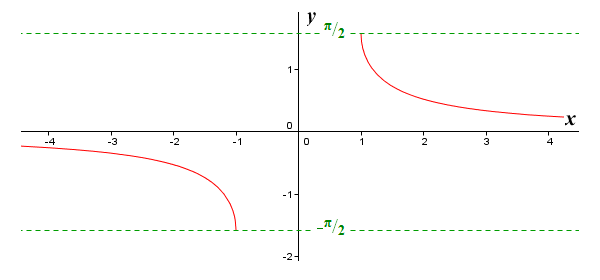The graph of y = arccsc (x) for -π/2 ≤ y ≤ π/2, except y = 0